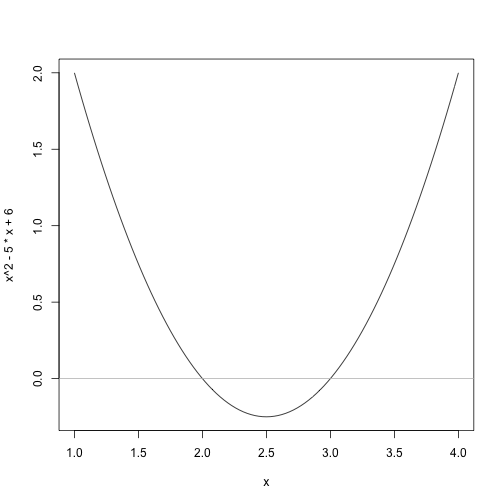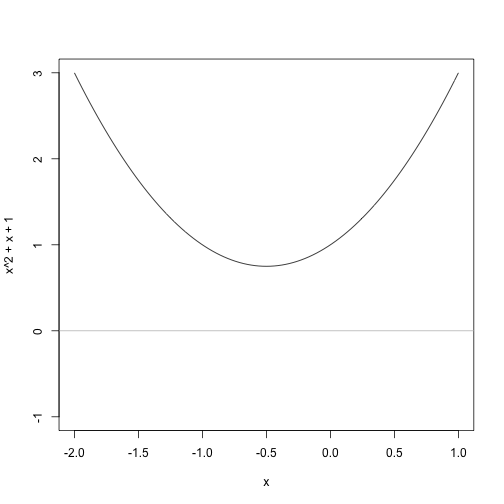The teaching of functions generally follows by showing various families of functions. The first is usually linear functions, or

$y = f(x) = mx+b$

These are simple functions and the basis for much of mathematics. They fit within the larger family of polynomial functions which have this form:

$f(x) = a_0 + a_1 x + a_2x^2 + a_3 x^3 + ... + a_n x^n$
where the $$a_0, a_1, ..., a_n$$ are the coefficients. The linear equation has $$a_2 = a_3 = ... = a_n = 0$$, so it is of this type. (These are polynomials of degree n, linear functions have degree 1.)

Now after linear functions, the next easiest are the quadratic funtions:

$f(x) = a_0 + a_1 x + a_2x^2 = ax^2 + bx + c$
The latter using the more familar names for the 3 coefficients. We assume $$a$$ is not 0, as otherwise we will not have a quadratic.

• The graphs are “U” shaped. They point up if $$a> 0$$, down if $$a < 0$$ and are lines when $$a = 0$$.

• The graphs may or may not intersect the x axis

• The graphs are “parabolic”

The 2nd point is what I want to talk about. When does the graph of a function intersect the x axis? Well, this is when

$f(x) = 0$

For quadratic functions you can solve this two ways:

1) you can factor (sometimes) and get $$f(x) = a(x-r)(x-s)$$, in which case r and s are the solutions, as $$a(x-r)(x-s)=0$$ when $$a = 0$$ or $$(x-r)=0$$ or $$(x-s) = 0$$ and no other ways. (If real numbers multiply to 0 then one or more must be 0.)

For example, if $$f(x) = x^2 - 5x + 6$$ then we have $$f(x) = (x-3)(x-2)$$ and both 3 and 2 are solutions. The graph shows this, as this is where it crosses the x axis:

curve(x^2 - 5 * x + 6, 1, 4)
abline(h = 0, col = "gray")2) You may not be able to factor. But that is because in high school we are taught to factor over the integers only. (Meaning the answer is nice). In fact, there is this fundamental theorem of algebra that says an $$n$$ degree polynomial will have $$n$$ roots if you count right (some may be repeated, some may be complex numbers but not real numbers). So a quadratic equation will always have 2 roots, though they may repeat $$(x^2 = 0)$$ or be complex (e.g., $$x^2 = -1$$).

In general, finding the roots of a polynomial (where it crosses the x axis) is not always solvable (Galois showed this), for quadratics they are, as was known by the Greeks. (For 3rd and 4th degree polynomials it took much longer to find the trick, and 5 or higher has no general mechanism.). The quadratic equation is what works for us. It says that the roots of $$f(x) = ax^2 + bx + c$$ are given by

$\frac{-b \pm \sqrt{b^2 - 4ac}}{2a}$

The part $$b^2 - 4ac$$ is called the discriminant. It discriminates between the cases: no real roots (when it is is negative), a repeated root (when 0), and two real roots (when positive). Notice roots come in pairs – that is the +/- bit.

Okay, lets see that is works:

a <- 1
b <- -5
c <- 6
(-b + c(-1, 1) * sqrt(b^2 - 4 * a * c))/(2 * a)

##  2 3


Here is another one: $$f(x) = x^2 + x - 1$$. Notice it doesn't factor over the reals. (Try it if you want.) Does it have real roots? Yes because $$b^2 - 4ac = 1 -(-4) = 5 > 0$$. The answers are $$(-1 + \sqrt{5})/2$$ and $$(-1 - \sqrt{5})/2$$:

a = 1
b = 1
c = -1
(-b + c(-1, 1) * sqrt(b^2 - 4 * a * c))/(2 * a)

##  -1.618  0.618


Here is one where there are no real roots, $$f(x) = x^2 + x + 1$$:

a = 1
b = 1
c = +1
(-b + c(-1, 1) * sqrt(b^2 - 4 * a * c))/(2 * a)

## Warning message: NaNs produced

##  NaN NaN

curve(x^2 + x + 1, -2, 1, ylim = c(-1, 3))
abline(h = 0, col = "gray")